# SCC Education

## Maths in hindi algebra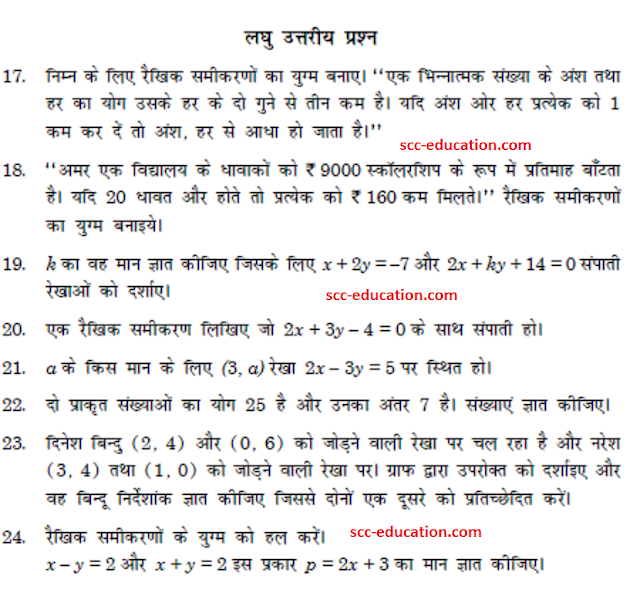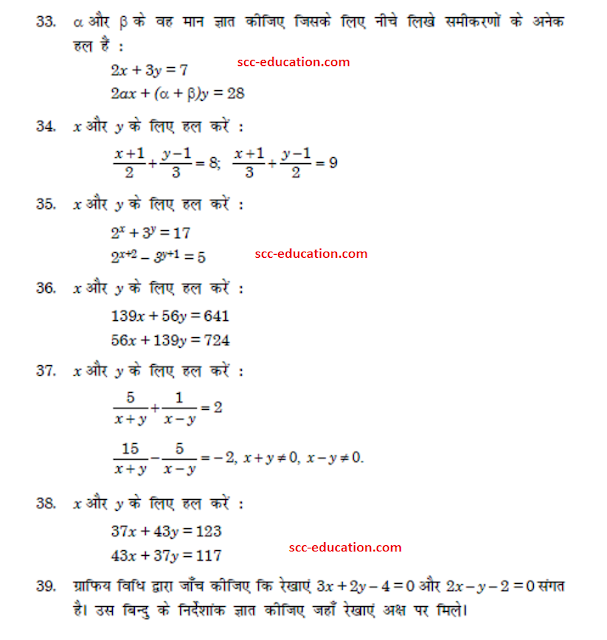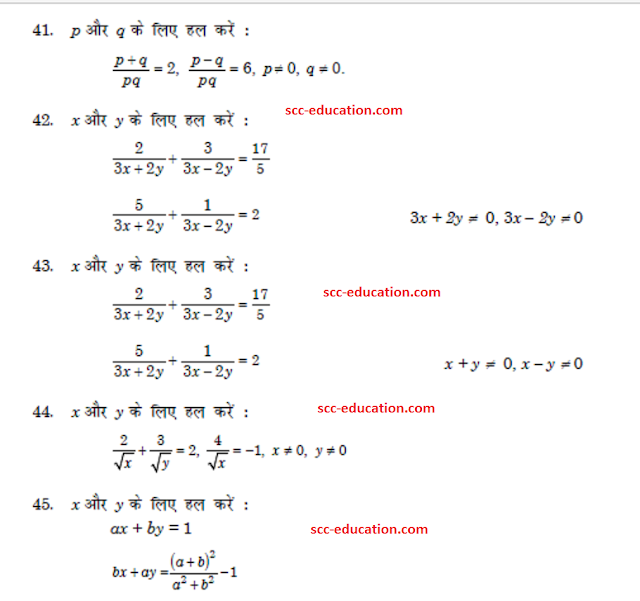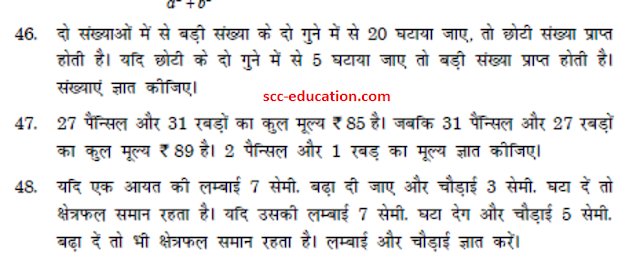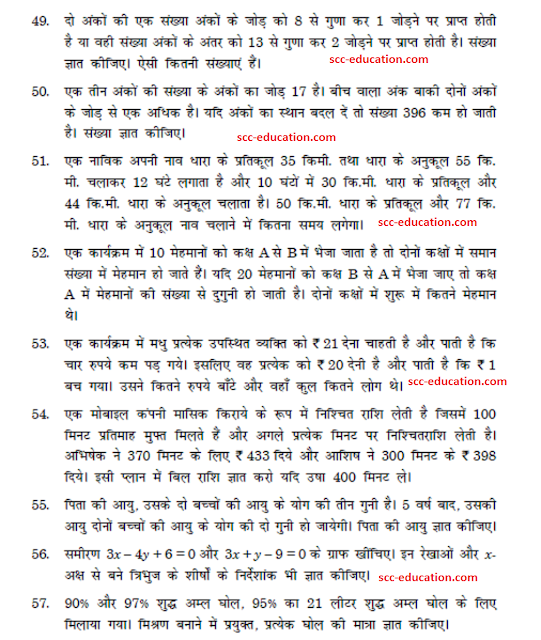Basic-trigonometry-identities

graphing-linear-inequalities solving

Introduction-of-co-ordinate-geometry

probability

materials-metals-and-non-metals

Trignometry-solved-questions

surface-areas-and-volumes

sequence

linear-equations

Basic-trigonometry-identities

Area of shaded region

Introduction-of-co-ordinate-geometry

Probability

Materials-metals-and-non-metals

Trignometry-solved-questions

Surface-areas-and-volumes

Sequence

Linear-equations

Sample paper 1

Sample paper 2

Sample paper 3

Sample paper 4

Circle (Important Question)

Arithmetic Progression

circle (hindi)

Probability  (hindi)

### Polynomial in hindi